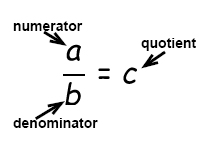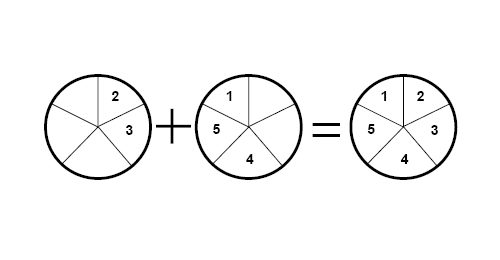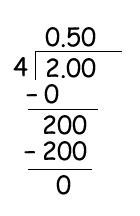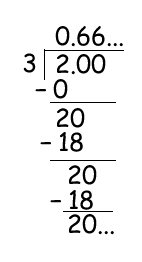# Calculating with decimals and fractions

Earlier you've learned how to calculate integers, now it's time to learn how to calculate decimals.

When you are calculating with decimal numbers you are using the same principles as when you are calculating with integers.

Example

$13.6 + 6.4=$

$=13+6+0.6+0.4=$

$= 19+1=20$

with variables

$2.6x + 3.8x=$

$=2x+3x+0.6x+0.8x =$

$=5x+1.4x=6.4x$

Decimal numbers are rational numbers and can be written as a fraction of two numbers.

We name fractions like this:Two fractions that are on the same denominator are called like fractions.

It is easy to add or subtract like fractions. If we add like fractions we just add the numerators and this sum will be the new numerator while the denominator is stays the same.

Example

$\frac{2}{5}+\frac{3}{5}= \frac{2+3}{5}=\frac{5}{5}$$\frac{4}{9}-\frac{2}{9}= \frac{4-2}{9}=\frac{2}{9}$

Factors that are not on the same denominator are called unlike fractions. To find the sum or the difference of unlike fractions we need to find a common denominator, the least common denominator - LCD.

To find the common denominator we multiply the first fraction by the denominator of the second fraction.

Example

$\frac{3}{4}+\frac{2}{3}=$

$LCD=4\cdot 3=12$

$\frac{3\cdot 3}{12}+\frac{2\cdot 4}{12}=\frac{9}{12}+\frac{8}{12}=$

$\frac{9+8}{12}=\frac{17}{12}$

You can rewrite fractions into decimal numbers by using long division.

Example

Rewrite 2/4 as decimals.This means that 2/4 is equal to 0.5. Decimals like 0.5 are called terminating decimals.

Example

Rewrite 2/3 as decimals.3 is not a factor of 2. 3 is not a factor of 20 but a factor of 18 which gives us a remainder of 2.

When you multiply fractions, the numerators will be multiplied with each other and the denominators will be multiplied with each other.

$\frac{x}{y}\cdot \frac{a}{b}=\frac{x\cdot a}{y\cdot b}=\frac{xa}{yb}$

Example

$\frac{2}{6}\cdot \frac{5}{8}=\frac{2\cdot 5}{6\cdot 8}=\frac{10}{48}\, \: or\: \, \frac{5}{24}$

When you are dividing fractions, you are going to multiply the first fraction by the multiplicative inverse of the second fraction.

$\frac{x}{y}\div \frac{a}{b}=\frac{x}{y}\cdot \frac{b}{a}=\frac{xb}{ya}$

$\frac{a}{b}\rightarrow \frac{b}{a}=\, Multiplicative \, inverse$

Example

$\frac{2}{6}\div \frac{5}{8}=\frac{2}{6}\cdot \frac{8}{5}=\frac{16}{30}\, \: or\: \, \frac{8}{15}$

## Video lesson

Rewrite into decimals